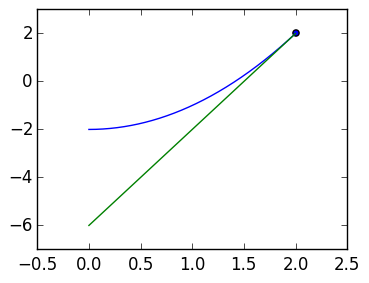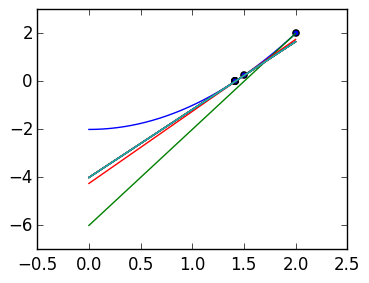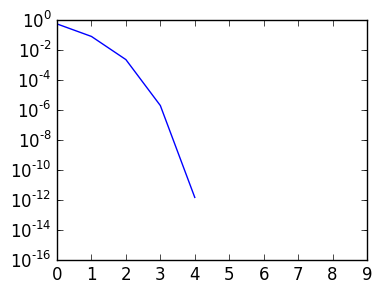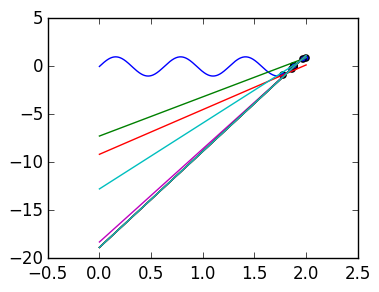# Lecture 38: Nonlinear problems¶

Suppose we want to solve a nonlinear ODE

$$u'(t) = f(t,u), u(0) = c$$

We saw already the forward Euler method:

$$w_{k+1}= w_k + h f(t_k,w_k)$$

where $w_0 = c$ and $t_k =k h$. This is an explicit method, because we get the value at $w_{k+1}$ explicitely from the previous value $w_k$.

If we try to implement backward Euler method, we get

$$w_{k+1} - h f(t_{k+1},w_{k+1}) = w_k$$

This is an implicit method, since the next value is implicitely defined. To solve this, we need to do root finding, that is, solve $g(w_{k+1}) = 0$ for

$$g(x) = x - h f(t_{k+1},x) - w_k$$

## Newton iteration¶

Suppose we want to solve

$$g(r) = 0$$

for $g(x) = x^2-2$. We can do so with Newton iteration: start at an guess $x_0=2$, approximating $g(x)$ by the tangent line at the point $g(x_0)$:

In :
using PyPlot

g=x->x.^2-2
gp=x->2x

p=linspace(0.,2.,1000)

x=zeros(1000)
x=2.

# g'(x)*(x-x)+g(x)

# g'(x)*(x-x)+g(x)  ==0
# x  ==x - g(x)/g'(x)

N=1
for k=1:N-1
x[k+1]=x[k]-g(x[k])/gp(x[k])
end

scatter(x[1:N],g(x[1:N]))

plot(p,g(p))
for k=1:N
plot(p,gp(x[k])*(p-x[k])+g(x[k]))
end

x[N]-sqrt(2.)Out:
0.5857864376269049

Finding the root of this line gives us the second guess

$$x_1 = x_0 - {f(x_0) \over f'(x_0)}$$

We can then repeat the process: approximate through the tangent line at $x_k$, then find the root

$$x_{k+1} = x_k - {f(x_k) \over f'(x_k)}.$$

This converges remarkably quickly:

In :
using PyPlot

g=x->x.^2-2
gp=x->2x

p=linspace(0.,2.,1000)

x=zeros(1000)
x=2.

# g'(x)*(x-x)+g(x)

# g'(x)*(x-x)+g(x)  ==0
# x  ==x - g(x)/g'(x)

N=10
for k=1:N-1
x[k+1]=x[k]-g(x[k])/gp(x[k])
end

scatter(x[1:N],g(x[1:N]))

plot(p,g(p))
for k=1:N
plot(p,gp(x[k])*(p-x[k])+g(x[k]))
end

x[N]-sqrt(2.)Out:
0.0

The following plot emonstrates that it converges faster than exponentially:

In :
err=abs(x[1:N]-sqrt(2.))
semilogy(err);For non-convex functions, the root it converges to (if any) is not predictible:

In :
g=x->sin(10x)
gp=x->10cos(10x)

p=linspace(0.,2.,1000)

x=zeros(1000)
x=2.

# g'(x)*(x-x)+g(x)

# g'(x)*(x-x)+g(x)  ==0
# x  ==x - g(x)/g'(x)

N=10
for k=1:N-1
x[k+1]=x[k]-g(x[k])/gp(x[k])
end

scatter(x[1:N],g(x[1:N]))

plot(p,g(p))
for k=1:N
plot(p,gp(x[k])*(p-x[k])+g(x[k]))
end

sin(10x[N])Out:
-7.347880794884119e-16

We can prove this result using Taylor series:

\begin{align*} 0 &= f(r) = f(x_k) + f'(x_k) (x_k -r) + {f''(\zeta_k) \over 2} (x_k-r)^2 \cr & = f(x_k) + f'(x_k) \epsilon_k + {f''(\zeta_k) \over 2} \epsilon_k^2 \end{align*}

But we have

$$\epsilon_k = x_k -r = x_k - x_{k+1} + x_{k+1}-r = x_k - x_{k+1} +\epsilon_{k+1}$$

giving us

$$0 = f(x_k) + f'(x_k) (x_k - x_{k+1}) + f'(x_k)\epsilon_{k+1} + {f''(\zeta_k) \over 2} \epsilon_k^2 But be definition$$

0=f(x_k) + f'(x_k) (xk - x{k+1})$$Thus we have$$\epsilon_{k+1} = - {f''(\zeta_k) \over 2 f'(x_k)} \epsilon_k^2

This is called quadratic convergence, as the error is roughly the previous error squared. Bounding derivatives can be used to get rigorous bounds on the convergence, and guaranteed convergence.